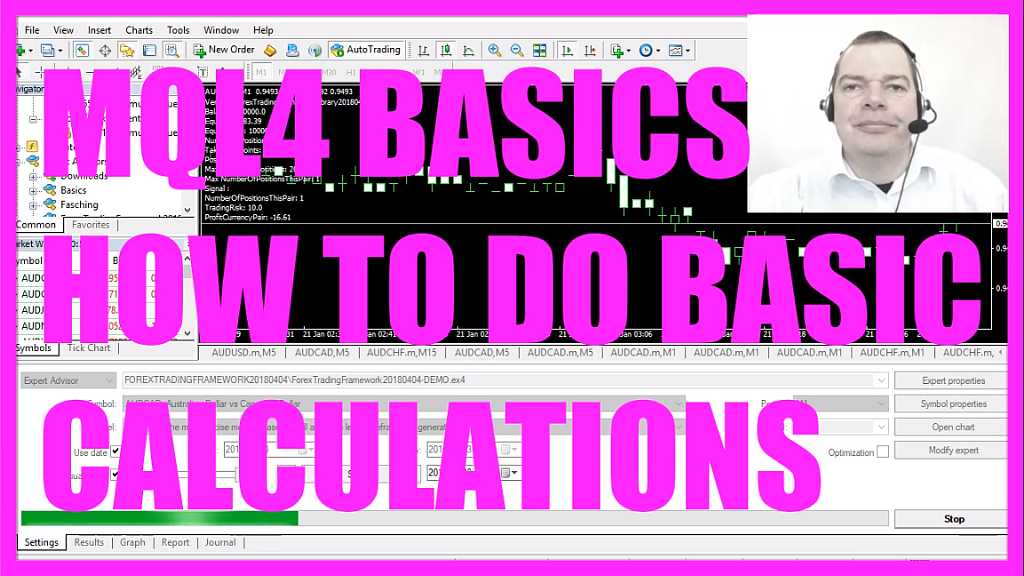In this video we want to talk about simple calculations, obviously whenever you use an automated program like this Expert Advisor you need to do calculations.
For example in our case we calculate the equity or the max number of allowed positions, so let’s find out how to do that.
First we need to click on the little icon here or press F4 to bring up Metaeditor and in Metaeditor we click on: “File/ New/ Expert Advisor (template)” from template, “Continue”, I will call this file: “SimpleCalculations”, click on “Continue”, “Continue” and “Finish” and now you can remove everything above the “OnTick” function and let’s also delete the two comment lines here.
So let’s start by defining two integer variables, they should be 5 and “b” should be 3, and let’s use the “Comment” function to output: ”The result is:” followed by the calculation.
In our case we start with “a” plus “b” and that’s it, when you’re done you can click on the “Compile“ button or press F7, you shouldn’t get any error messages here and if that is the case you can click here or press F4 to go back to Metatrader.
In Metatrader you want to click on: “View/ Strategy Tester” or press CTRL and R, let’s pick the new file: “SimpleCalculations.ex4”, enable the visualization and start the test.
And now we see the text: “The result is: 8” because 5 plus 3 is 8, let’s try a minus “b”, recompile the code, stop the test and start a new one, and now the result is 2 because 5 minus 3 equals 2.
We can also try to multiply “a” and “b”, so now it’s 5 times 3 and that of course equals 15.
So far so good, let’s try “a” divided by “b” and this time Metatrader says that the result is 1! – and the reason is that we have used integer variables so we can only deal with whole numbers. Let’s change the data type and use “double” and now we should get “The result is: 1.6667”. That is better!
Okay, what do you think is going on when we try to calculate this expression here?
“a” equals 5, 5 plus 5 would be 10, “b” equals 3, 3 times 10 would be 30, so let’s find out! – and our strategy tester says that the result is 20, and the reason is that the multiplication is calculated first, so that’s 5 times 3 and that equals 15 and afterwards “a” is added, and if you want to change the calculation order you can use round brackets and now you should get the result is 30 because everything within round brackets is calculated first and the part outside the brackets comes afterwards.
Okay, that’s it for the basic calculations.
If you open the MQL4 Reference by pressing F1 you will find lots of other math functions and it’s always a good idea to write such a small program here to find out how they work because in the future you are going to do more complex stuff and it will be helpful if you understand the basic stuff first because in this video you have learned how to do simple calculations and how to output the result on the chart and you have done it yourself with a few lines of MQL4 code.